# Triangle - math word problems

1. QuizQ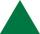An isosceles triangle has two sides of length 7 km and 39 km. How long is a third side?
2. HeightCalculate height of the equilateral triangle if its perimeter is 8?
3. Circumscribing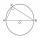Determine the radius of the circumscribed circle to the right triangle with legs 9 cm and 6 cm.
4. ClimbOn the road sign, which informs the climb is 8.7%. Car goes 5 km along this road. What is the height difference that car went?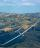Calculate how many g's (gravity accelerations) feel glider pilot when turning the horizontal circles of radius 148 m flying at 95 km/h. Centripetal acceleration is proportional to the square of the speed and inversely proportional to the radius of rotatio
6. SinesIn ▵ ABC, if sin(α)=0.5 and sin(β)=0.6 calculate sin(γ)
7. OK circleCalculate the radius (circumradius) of the circle described right triangle with hypotenuse long 33 and one cathetus long 17.
8. R TrapeziumRectangular trapezium has bases 12 and 5 and area 84 cm2. What is its perimeter?
9. AircraftThe plane flies at altitude 6500 m. At the time of first measurement was to see the elevation angle of 21° and second measurement of the elevation angle of 46°. Calculate the distance the plane flew between the two measurements.
10. ReflectorCircular reflector throws light cone with a vertex angle 49° and is on 33 m height tower. The axis of the light beam has with the axis of the tower angle 30°. What is the maximum length of the illuminated horizontal plane?
11. 4s pyramidRegular tetrahedral pyramid has a base edge a=17 and collaterally edge length b=32. What is its height?
12. It is rectangular?Size of two of the angles in a triangle are: α=110°, β=40°. Is it a right triangle?
13. Triangle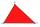For how many integer values of x can 16, 15 and x be the lengths of the sides of triangle?
14. Tetrahedral pyramidWhat is the surface of a regular tetrahedral (four-sided) pyramid if the base edge a=16 and height v=16?
15. Side cIn △ABC a=2, b=4 and ∠C=100°. Calculate length of the side c.
16. Two boatsTwo boats are located from a height of 150m above the surface of the lake at depth angles of 57° and 39°. Find the distance of both boats if the sighting device and both ships are in a plane perpendicular to the surface of the lake.
17. StairwayWhat angle rising stairway if step height in 17 cm and width 27 cm?
18. Prism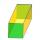The lenght, width and height of a right prism are 17, 11 and 11 respectively. What is the lenght of the longest segment whose endpoints are vertices of the prism?
19. Distance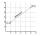Calculate distance between two points X[18; 19] and W[20; 3].
20. ObserverThe observer sees straight fence 100 m long in 30° view angle. From one end of the fence is 102 m. How far is it from the another end of the fence?

Do you have an interesting mathematical word problem that you can't solve it? Submit math problem, and we can try to solve it.

We will send a solution to your e-mail address. Solved examples are also published here. Please enter the e-mail correctly and check whether you don't have a full mailbox.

Please do not submit problems from current active competitions such as Mathematical Olympiad, correspondence seminars etc...